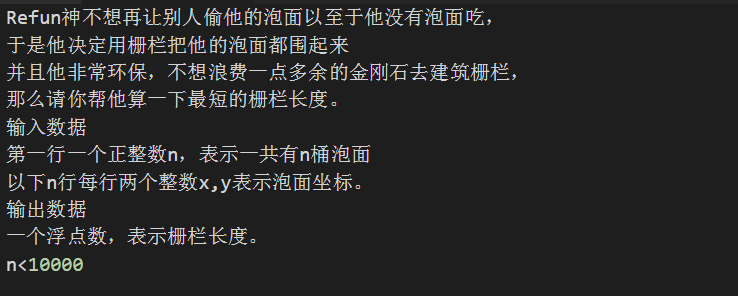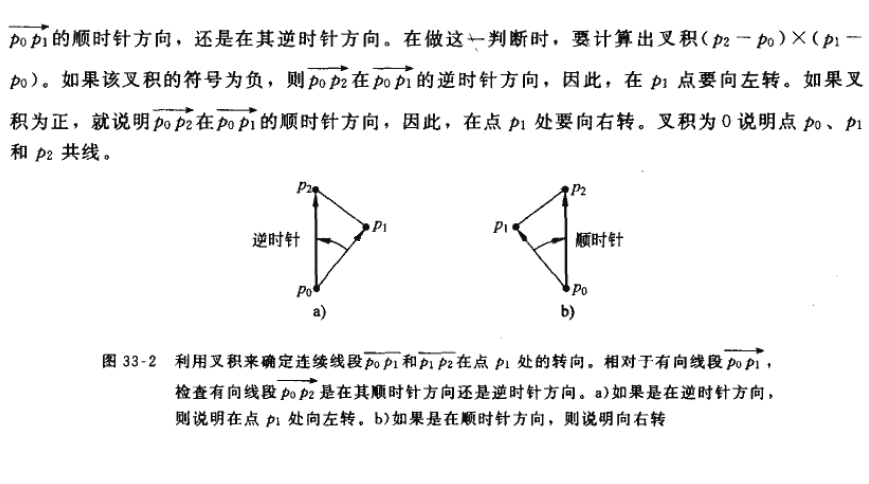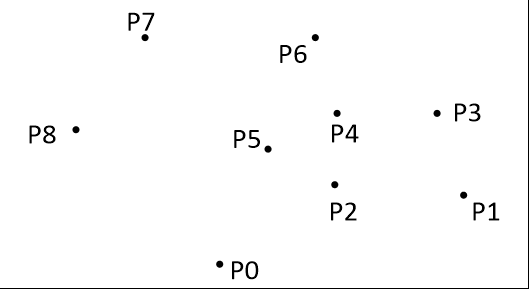# 凸包小结mhr:明显，这个题我们可以暴力枚举!

graham算法的整个操作基本都在一个栈中完成。如果设所有点的集合为点集Q，那么Q中的所有点都要入栈一次，然后再把一部分不符合要求的点弹出栈，最后剩在栈中的点就是凸包上的点了。

1. 首先我们要选取一个基点o，要求在点集Q中，基点o纵坐标必须是最小的，如果有相同最小的纵坐标，那么选取横坐标最小的，如果还有相同的。。肯定是重点，不用管它就是了。如何快速找到？如果你不嫌麻烦，大可以sort排序之后选第一个，然而实际上，我们只需要找出那个最下面同时是最左边的点就可以了，因为之后整个点集还会重新排序，所以这一开始的顺序没什么用。
2. 然后我们把剩下的所有点以o为极点，进行极角排序，角小的放在前面，如果角度相同，那么按照到点o的距离排序。

3. 设所有的点事p1,p2.....pn。将o，p1，p2三个点压入栈，开始遍历所有剩下的点。

4. 对每一个新遍历到的点，很明显我们需要逆时针旋转当前方向，如果有一个点顺时针旋转了，那么我们就把栈顶的点弹出，直到符合逆时针旋转这个要求为止。然而其实也有瑕疵，就是这个正负和你计算的顺序是有关系的，经常有不等号写反结果一直爆0的现象发生。所以有句老话“尽信书则不如无书”，不要过分相信书上说的，多实践才是真理。。

p.s：以上面那道题目为例。

#include<iostream>
#include<cstdio>
#include<cstring>
#include<algorithm>
#include<cmath>
#include<queue>
#define ll long long
#define inf 50000000
#define re register
#define MAXN 50005
using namespace std;
struct node{
double x,y;
};
node a[MAXN],stackk[MAXN];
double xx,yy;
int n,top;
{
int x=0,c=1;
char ch=' ';
while((ch>'9'||ch<'0')&&ch!='-')ch=getchar();
while(ch=='-') c*=-1,ch=getchar();
while(ch<='9'&&ch>='0')x=x*10+ch-'0',ch=getchar();
return x*c;
}
inline double js(node a,node b)//计算距离自不必说
{
return sqrt((a.x-b.x)*(a.x-b.x)*1.0+(a.y-b.y)*(a.y-b.y)*1.0);
}
inline bool cmp(node a,node b)//第一遍排序，来求基点。
{
if(a.y==b.y) return a.x<b.x;
return a.y<b.y;
}
inline double cross(node a,node b,node c)//计算以a为公共端点，b与c的叉积。
{
return (b.x-a.x)*(c.y-a.y)-(c.x-a.x)*(b.y-a.y);
}
inline bool cmp1(node a,node b)//极角排序
{
double k=cross(stackk,a,b);
if(k>0) return 1;//如果b在a时针方向返回1
else if(k==0) return js(stackk,a)<=js(stackk,b);//如果极角相等，则比较距离
else return 0;
}
int main()
{
for(re int i=1;i<=n;i++){
scanf("%lf%lf",&a[i].x,&a[i].y);
}
if(n==1) {printf("0");return 0;}
if(n==2) {printf("%.2lf",js(a,a));return 0;}
sort(a+1,a+n+1,cmp);
stackk[++top]=a;
xx=stackk[top].x;
yy=stackk[top].y;
sort(a+2,a+n+1,cmp1);
stackk[++top]=a;
stackk[++top]=a;//把p1，p2，p3压入栈中。
for(re int i=4;i<=n;i++){
while(top>0&&cross(stackk[top-1],stackk[top],a[i])<0)//如果右旋转了，就弹出栈顶的点
top--;
stackk[++top]=a[i];//加入新点
}
double ans=0;
for(re int i=2;i<=top;i++)//点之间两两求距离。
ans+=js(stackk[i-1],stackk[i]);
ans+=js(stackk[top],stackk);
printf("%.2lf",ans);
}

posted @ 2018-03-28 20:37  ~victorique~  阅读(...)  评论(...编辑  收藏
Live2D